Question

Find an optimal parenthesization for matrix-chain multiplications using any PYTHON/java/c++/c for the number {26, 9, 41, 18, 13, 22, 28, 32, 25, 26, 30, 37, 19, 47, 11, 24, 20} using a top-down memorized solution. The output must be three lines:

1) the first line contains the optimal number of multiplication

2) the second line contains the optimal parenthesization and

3) the third line is the time required to compute the optimal parenthesization

//C++ program

#include<iostream>
#include <chrono>
#include <ctime>
#include<limits.h>
using namespace std;

void Parenthesis(int , int , int ,int *, char &) ;
void matrixChainMultiplication(int *, int );

int main()
{
int arr[] = {26, 9, 41, 18, 13, 22, 28, 32, 25, 26, 30, 37, 19, 47, 11, 24, 20};
int n = sizeof(arr)/sizeof(arr);
matrixChainMultiplication(arr, n);
return 0;
}

void matrixChainMultiplication(int arr[], int n)
{
int DP[n][n];
int bracket[n][n];
for (int i=1; i<n; i++)
DP[i][i] = 0;

for (int L=2; L<n; L++)
{
for (int i=1; i<n-L+1; i++)
{
int j = i+L-1;
DP[i][j] = INT_MAX;
for (int k=i; k<=j-1; k++)
{
int q = DP[i][k] + DP[k+1][j] + arr[i-1]*arr[k]*arr[j];
if (q < DP[i][j])
{
DP[i][j] = q;
bracket[i][j] = k;
}
}
}
}

cout<<"Optimal number of multiplication : "<<DP[n-1]<<"\n";
char name = 'A';

std::chrono::time_point<std::chrono::system_clock> start, end;
double time;
std::chrono::duration<double> elapsed_seconds;

cout << "Optimal Parenthesization is : ";
start = std::chrono::system_clock::now();
Parenthesis(1, n-1, n, (int *)bracket, name);
end = std::chrono::system_clock::now();
elapsed_seconds = end - start;
time= elapsed_seconds.count();

cout<<"\nTime required to compute the optimal parenthesization "<<time<<"\n\n";

}

void Parenthesis(int i, int j, int n,int *bracket, char &name)
{

if (i == j)
{
cout << name++;
return;
}

cout << "(";

Parenthesis(i, *((bracket+i*n)+j), n,bracket, name);

Parenthesis(*((bracket+i*n)+j) + 1, j,n, bracket, name);
cout << ")";
}
//sample output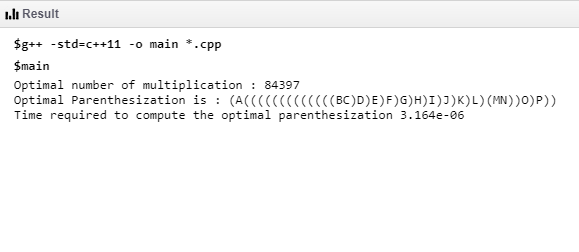#### Earn Coins

Coins can be redeemed for fabulous gifts.

Similar Homework Help Questions
• ### Find an optimal parenthesization for matrix-chain multiplications using any language PYTHON/Java/C++ ,C for the number {26, 9, 41, 18, 13, 22, 28, 32, 25, 26, 30, 37, 19, 47, 11, 24, 20} using a strai...

Find an optimal parenthesization for matrix-chain multiplications using any language PYTHON/Java/C++ ,C for the number {26, 9, 41, 18, 13, 22, 28, 32, 25, 26, 30, 37, 19, 47, 11, 24, 20} using a straight forward-recursive solution. The output must be three lines: 1) the first line contains the optimal number of multiplication 2) the second line contains the optimal parenthesization and 3) the third line is the time required to compute the optimal parenthesization

• ### Iwant answer this using number. Airthemitics. Varibles by python This using if else in python The...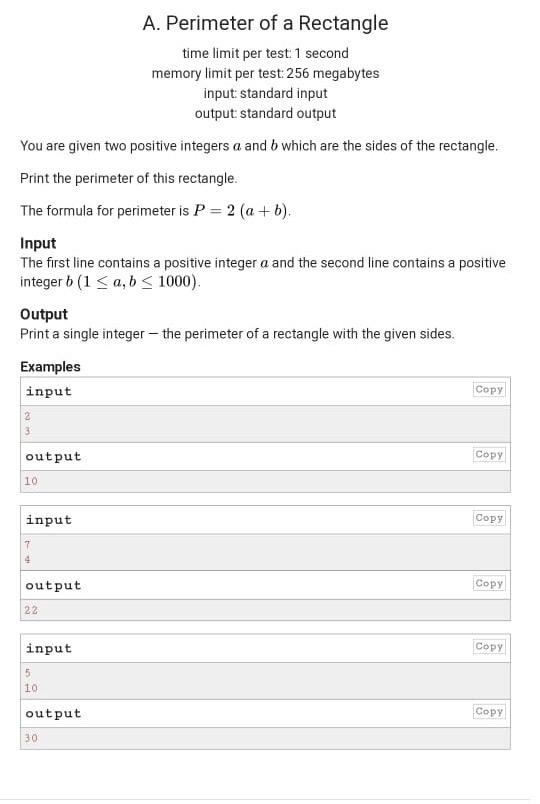Iwant answer this using number. Airthemitics. Varibles by python This using if else in python The third picture using if. Elif. Else This using for loop in python A. Perimeter of a Rectangle time limit per test 1 second memory limit per test: 256 megabytes input standard input output standard output You are given two positive integers a and b which are the sides of the rectangle. Print the perimeter of this rectangle. The formula for perimeter is P =...

• ### Write a Java program, In this project, you are going to build a max-heap using array...

Write a Java program, In this project, you are going to build a max-heap using array representation. In particular, your program should: • Implement two methods of building a max-heap. o Using sequential insertions (its time complexity: ?(?????), by successively applying the regular add method). o Using the optimal method (its time complexity: ?(?), the “smart” way we learned in class). For both methods, your implementations need to keep track of how many swaps (swapping parent and child) are required...

• ### Magic Square question for Python

You have this solution in Java, I am interested in this same solution for Python.One interesting application of two-dimensional arrays is magic squares. A magic square is a square matrix in which the sum of every row, every column, and bothdiagonals is the same. Magic squares have been studied for many years, and there are some particularly famous magic squares. In this exercise you will write code todetermine whether a square is magic.You should find that the first, second, and...

• ### I need help using python ( spyder) only use files , loops if needed is statement do not use , def...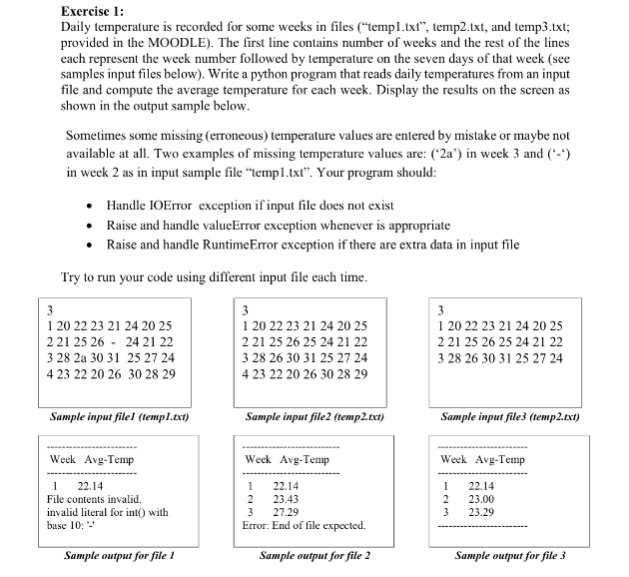i need help using python ( spyder) only use files , loops if needed is statement do not use , def function or any other. Exercise 1: Daily temperature is recorded for some weeks in files (templ.txt", temp2.txt, and temp3.txt; provided in the MOODLE). The first line contains number of weeks and the rest of the lines each represent the week number followed by temperature on the seven days of that week (see samples input files below). Write a python...

• ### C# 1. Given two lengths between 0 and 9, create an rowLength by colLength matrix with...

C# 1. Given two lengths between 0 and 9, create an rowLength by colLength matrix with each element representing its column and row value, starting from 1. So the element at the first column and the first row will be 11. If either length is out of the range, simply return a null. For exmaple, if colLength = 5 and rowLength = 4, you will see: 11 12 13 14 15 21 22 23 24 25 31 32 33 34...

• ### C# Visual Studio using System; using System.Collections.Generic; using System.Text; 1. Modify the program to output that...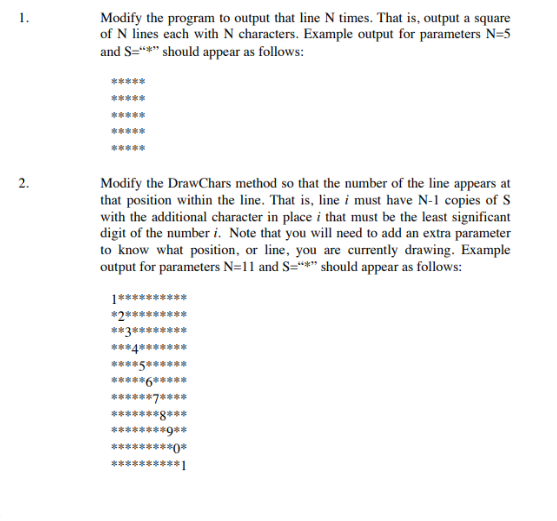C# Visual Studio using System; using System.Collections.Generic; using System.Text; 1. Modify the program to output that line N times. That is, output a square of N lines each with N characters. Example output for parameters N=5 and S="*" should appear as follows: 多多本事事 串串串串串 事事事串串 事事事事事 2. Modify the DrawChars method so that the number of the line appears at that position within the line. That is, line i must have N-1 copies of S with the additional character in...

• ### Objective : Write a C Shell script which copies all files(*.java and *.class) from your home dire...

Objective : Write a C Shell script which copies all files(*.java and *.class) from your home directory to a new one, and Analyze the new directory information such as number of files, user permissions, and disk usage. Sample Output:                                                    << CS Directory Analysis >>      Date:   ============================================================ Current Directory: /home/tomss New Directory Created : /home/tomss/pgm01 File information Total Number of files : 22 files Directory files:   0 files Plain text files:   10 files File have read permissions: 3 files File have...

• ### Python 1 import matplotlib.pyplot as plt 2 import numpy as np 3 4 abscissa = np.arange(20) 5 plt....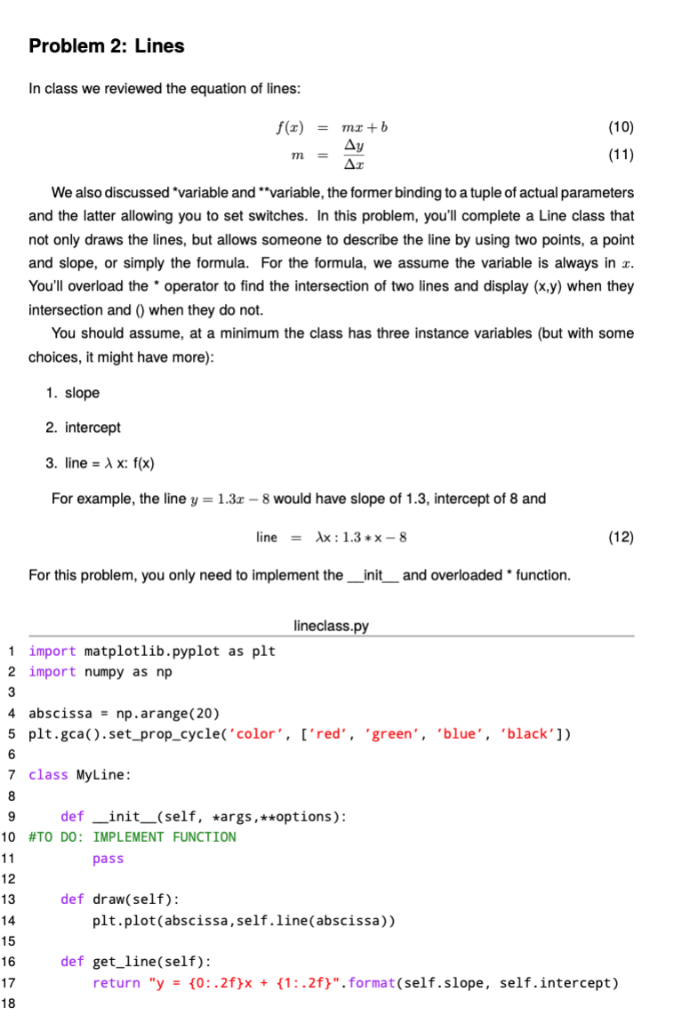python 1 import matplotlib.pyplot as plt 2 import numpy as np 3 4 abscissa = np.arange(20) 5 plt.gca().set_prop_cycle( ’ color ’ , [ ’ red ’ , ’ green ’ , ’ blue ’ , ’ black ’ ]) 6 7 class MyLine: 8 9 def __init__(self, * args, ** options): 10 #TO DO: IMPLEMENT FUNCTION 11 pass 12 13 def draw(self): 14 plt.plot(abscissa,self.line(abscissa)) 15 16 def get_line(self): 17 return "y = {0:.2f}x + {1:.2f}".format(self.slope, self.intercept) 18 19 def __str__(self):...

• ### // This program displays a menu and asks the user to make a 2 // selection....

// This program displays a menu and asks the user to make a 2 // selection. An if/else if statement determines which item 3 // the user has chosen. 4 #include <iostream> 5 #include <iomanip> 6 using namespace std; 7 8 int main() 9 { 10 int choice; // To hold a menu choice 11 int months; // To hold the number of months 12 double charges; // To hold the monthly charges 13 14 // Constants for membership rates...